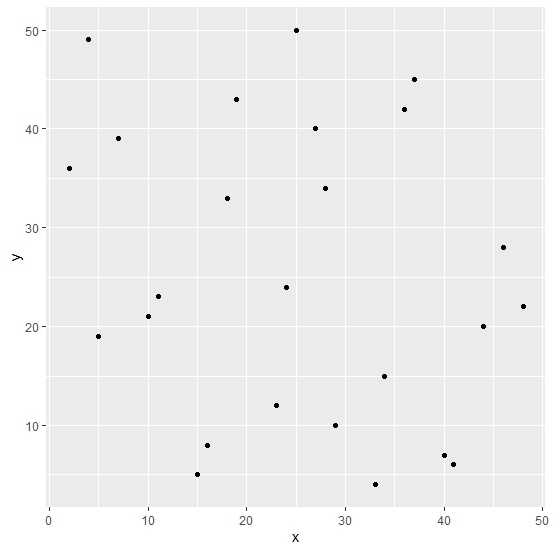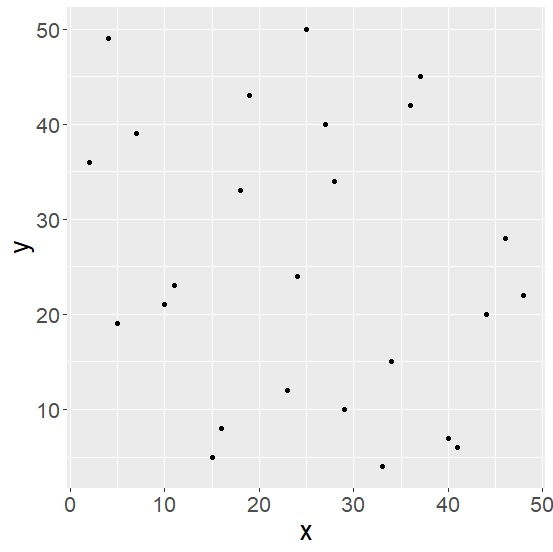# How to make all text size same in a plot created by using ggplot2 in R?

R ProgrammingServer Side ProgrammingProgramming

To make all text size same in a plot created by using ggplot2 in R, we can follow the below steps −

• First of all, create a data frame.
• Then, create a chart using ggplot2.
• After that, create the same chart with theme function and change the text size using text argument.

## Create the data frame

Let's create a data frame as shown below −

Live Demo

x<-sample(1:50,25)
y<-sample(1:50,25)
df<-data.frame(x,y)
df

On executing, the above script generates the below output(this output will vary on your system due to randomization) −

  x   y
1 25 50
2 46 28
3 29 10
4 16 8
5 37 45
6 41 6
7 27 40
8 28 34
9 33  4
10 10 21
11 40 7
12 36 42
13 18 33
14 11 23
15 5  19
16 24 24
17 23 12
18 48 22
19 7  39
20 4  49
21 19 43
22 15 5
23 2  36
24 44 20
25 34 15

## Create a plot using ggplot2

Using geom_point to create scatterplot between x and y −

x<-sample(1:50,25)
y<-sample(1:50,25)
df<-data.frame(x,y)
library(ggplot2)
ggplot(df,aes(x,y))+geom_point()

### Output## Create the above plot with same text size

Adding theme function to the above script and setting text size to 20 for all the text shown in the graph −

x<-sample(1:50,25)
y<-sample(1:50,25)
df<-data.frame(x,y)
library(ggplot2)
ggplot(df,aes(x,y))+geom_point()+theme(text=element_text(size=20))

### Output